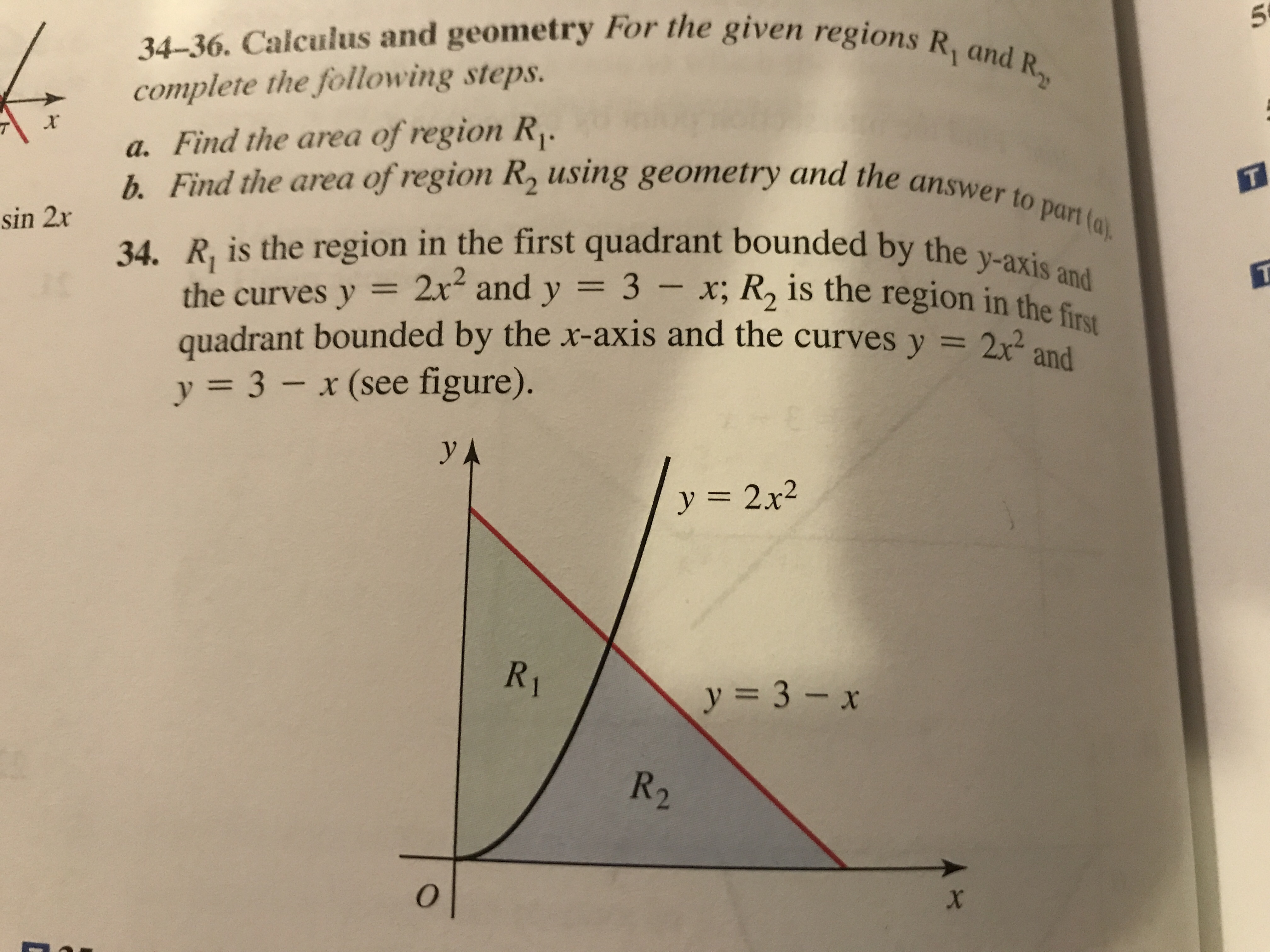# en regions R and R 34-36. Calculus and geometry For the given regions complete the following steps. a. Find the area of region R b. Find the area of'region R using geometry and the answerto 234. R, is the region in the first quadrant bounded by the part (o unded by the y-axis and 2r2 and y 3- x; R2 is the region in the firy quadrant bounded by the x-axis and the curves y = 2x2 y-: 3-x (see figure) and y 2x2

Question

#34help_outlineImage Transcriptioncloseen regions R and R 34-36. Calculus and geometry For the given regions complete the following steps. a. Find the area of region R b. Find the area of'region R using geometry and the answerto 234. R, is the region in the first quadrant bounded by the part (o unded by the y-axis and 2r2 and y 3- x; R2 is the region in the firy quadrant bounded by the x-axis and the curves y = 2x2 y-: 3-x (see figure) and y 2x2 fullscreen